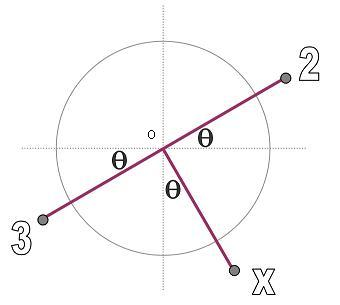#### You may also like### Coke Machine

The coke machine in college takes 50 pence pieces. It also takes a certain foreign coin of traditional design...### At a Glance

The area of a regular pentagon looks about twice as a big as the pentangle star drawn within it. Is it?### Cosines Rule

Three points A, B and C lie in this order on a line, and P is any point in the plane. Use the Cosine Rule to prove the following statement.

##### Age 14 to 16 Challenge Level:

Well done Shaun from Nottingham and Maria from Seville.In this diagram $OX$ makes an angle $\theta$ with the vertical, which means that the $2$ and $3$ weights both make an angle $\theta$ with the horizontal.

If we take the length $OX$ as the unit and then think about a vertical line through $O$ and the horizontal space between that line and vertical lines through the $2$ and $3$ weights, and also through $X$.

We can see that the horizontal shift from the pivot for $X$ is $\sin\theta$ and the horizontal shift from the pivot for the $2$ and for the $3$ is $\cos\theta$. The balance will come to a settled position so that:
$$3\cos\theta = 2\cos\theta + X\sin\theta$$
which means that $X\sin\theta$ must equal $\cos\theta$ or, after a little rearranging,
$$X = \frac{ \cos\theta}{ \sin\theta}\;.$$

At Stage 4 this equation is properly best solved by trial and improvement but if you have gone just a little bit further with your maths, you may know that:
$$\tan\theta = \frac{ \sin\theta}{ \cos\theta}\;.$$
Investigate that if you haven't seen it before.

So here $\tan\theta$ will equal $1/x$, and all we need to do is find $1/x$ on a calculator and then take the inverse Tangent for that value. In degrees the specific angles were $45$ degrees and $26.6$ degrees (to $1$ decimal place).